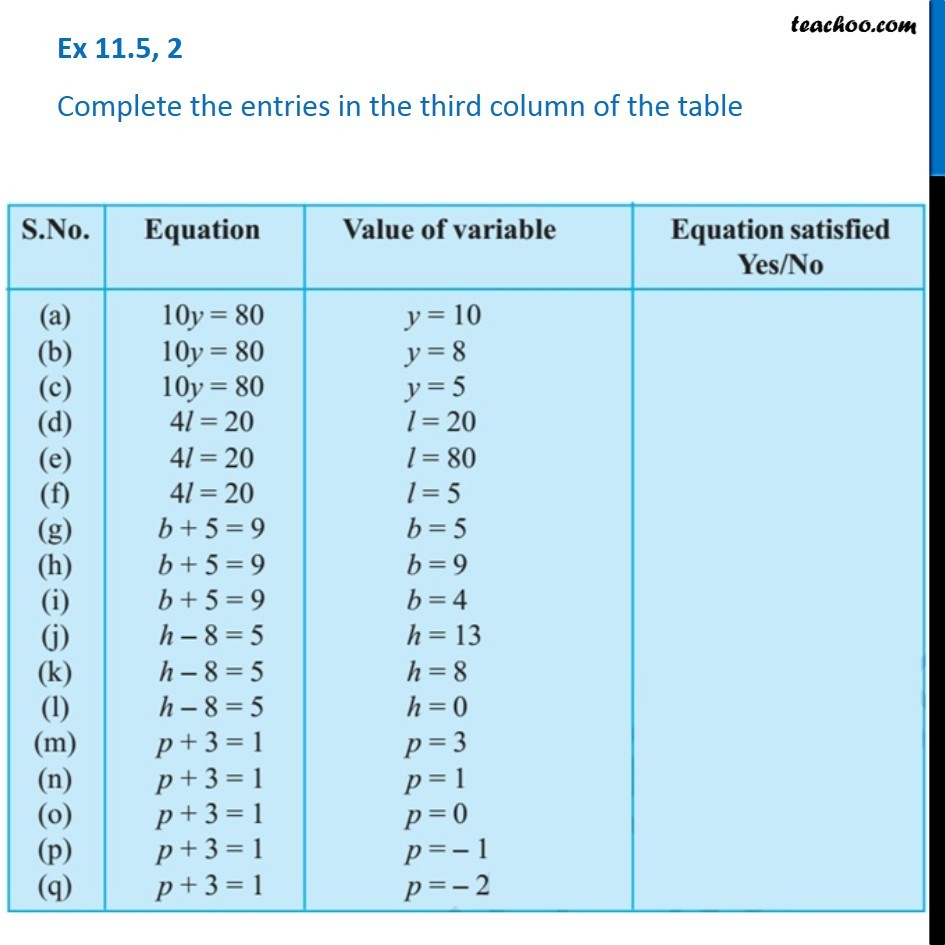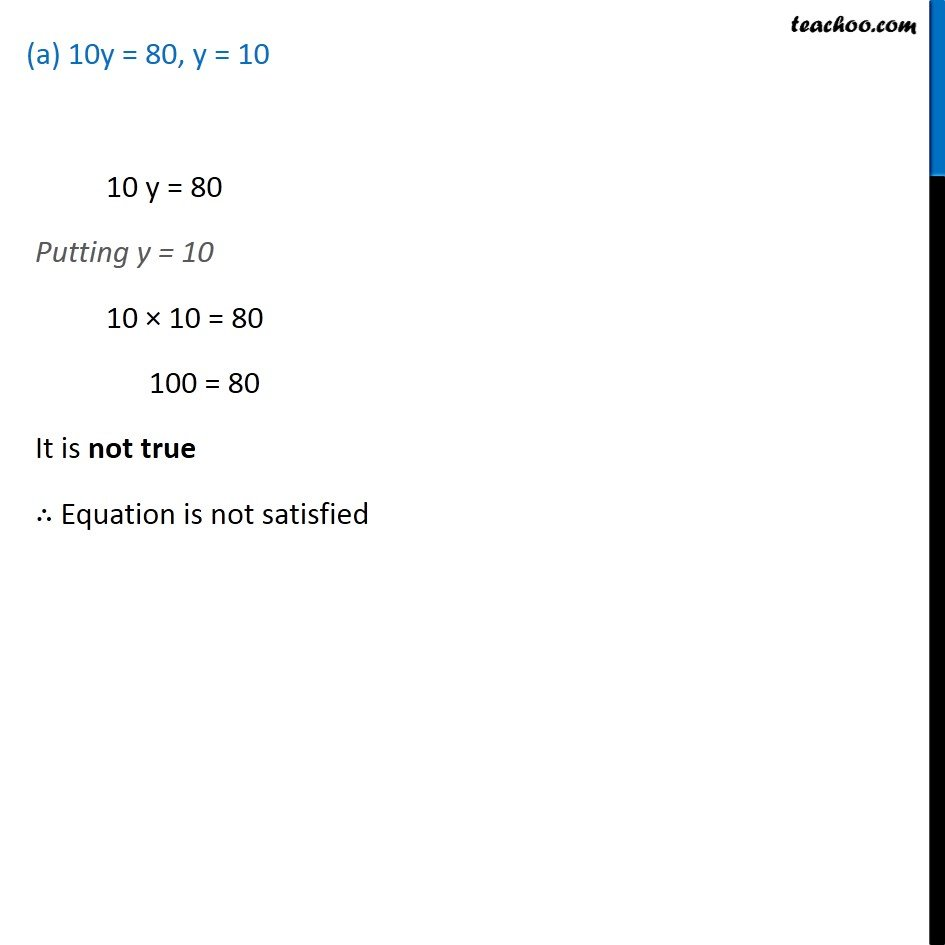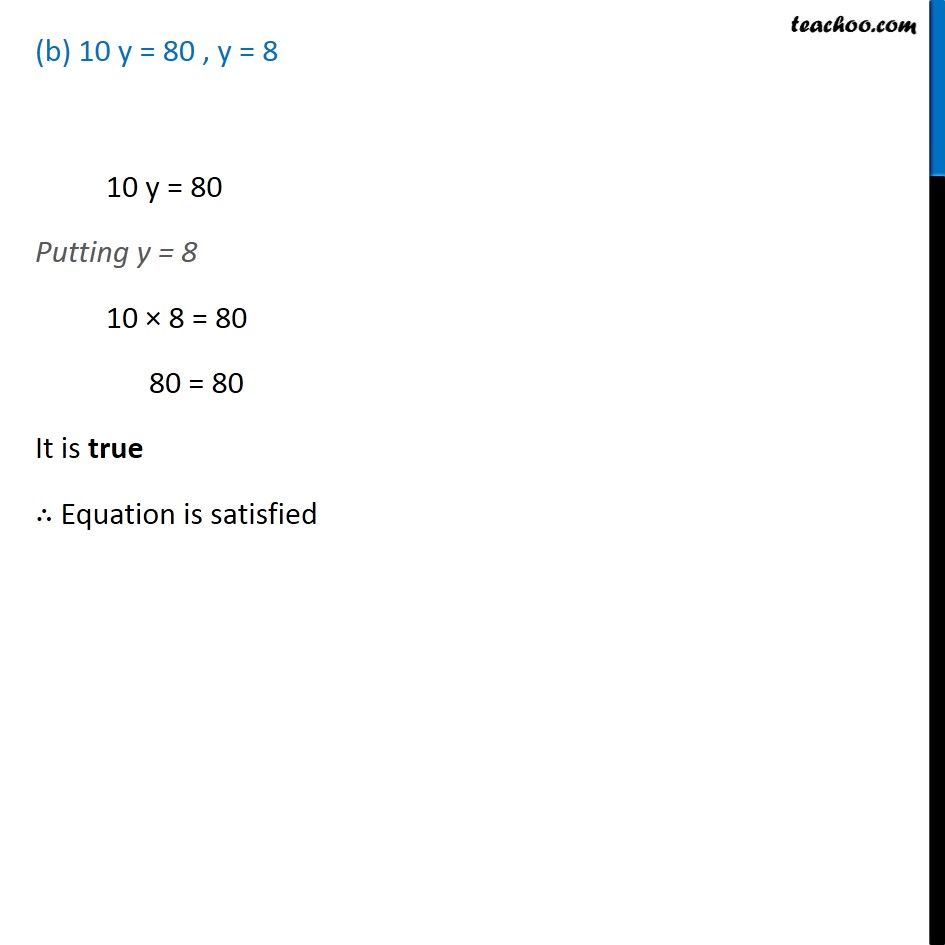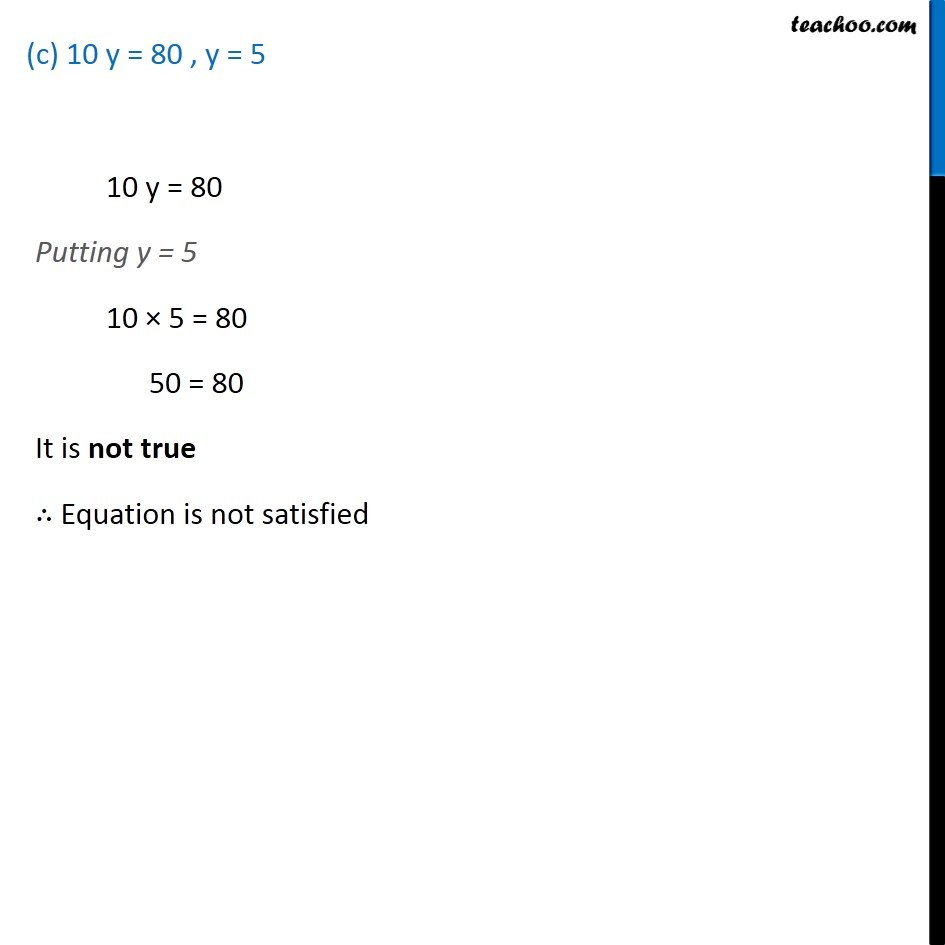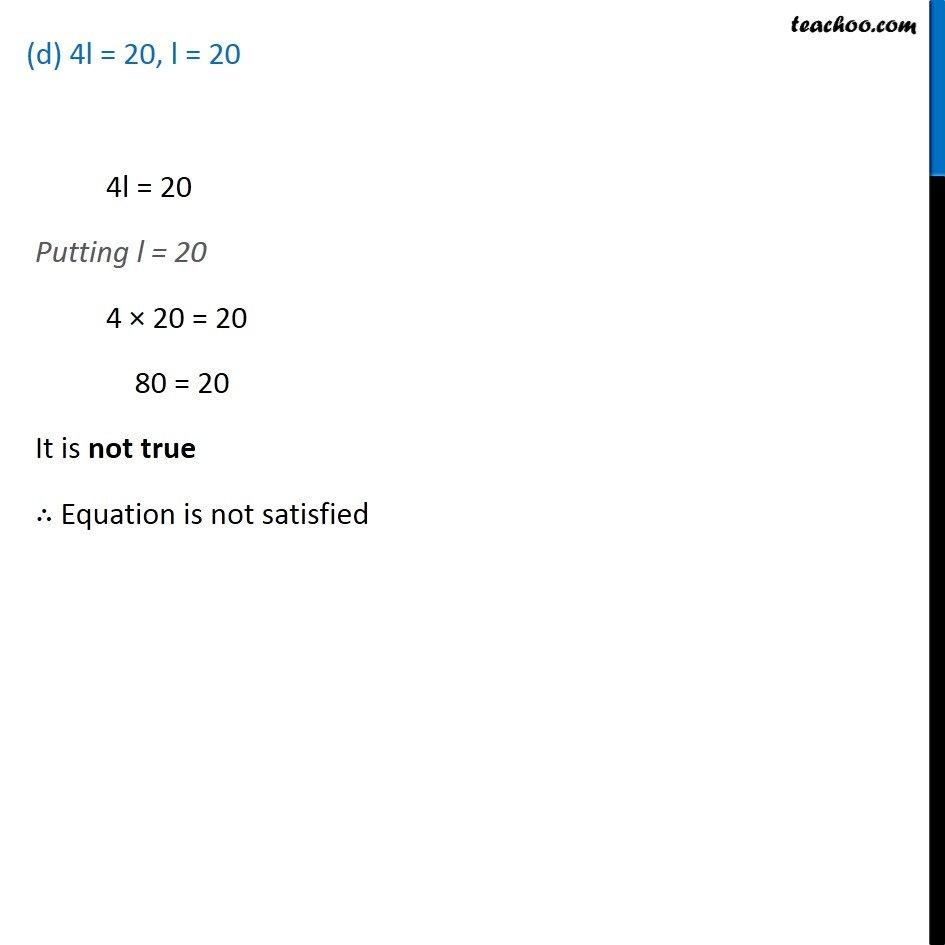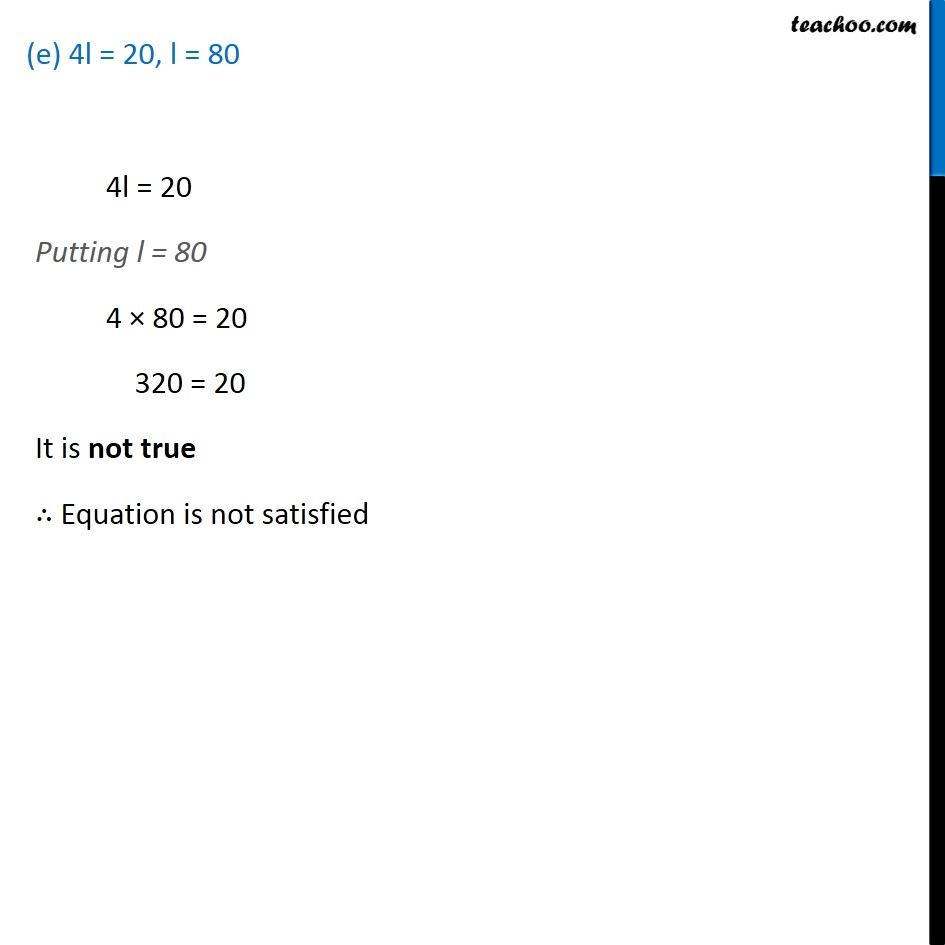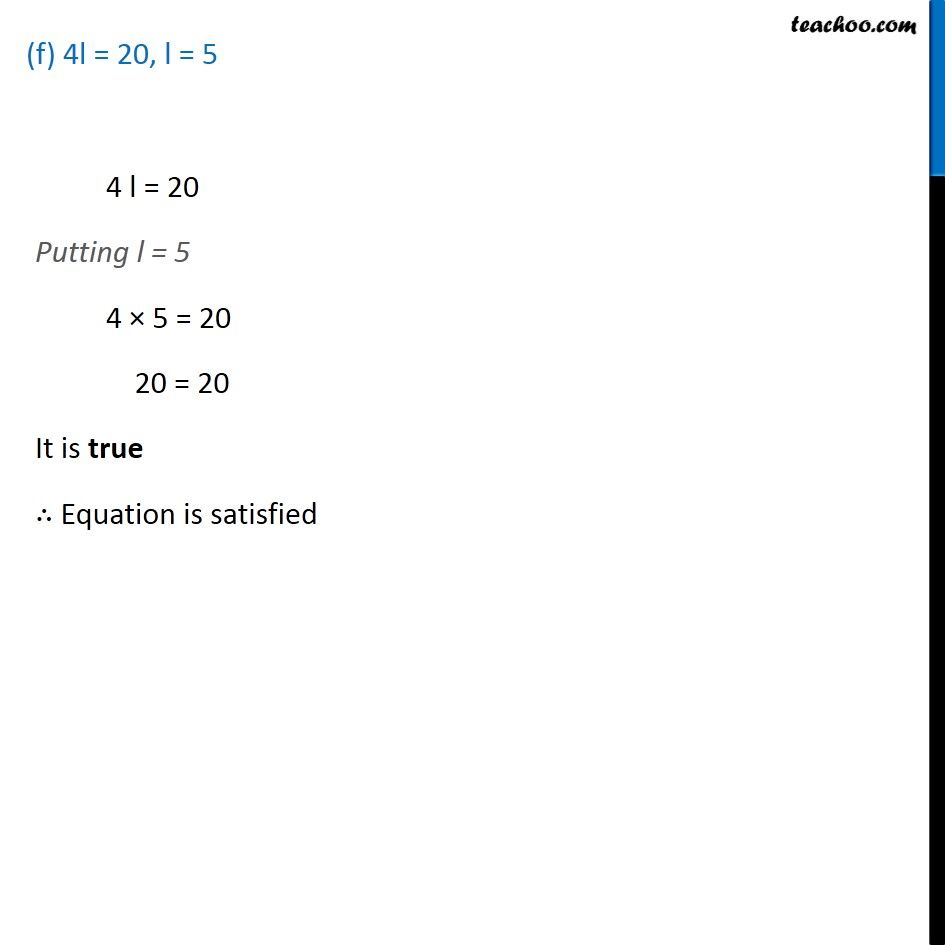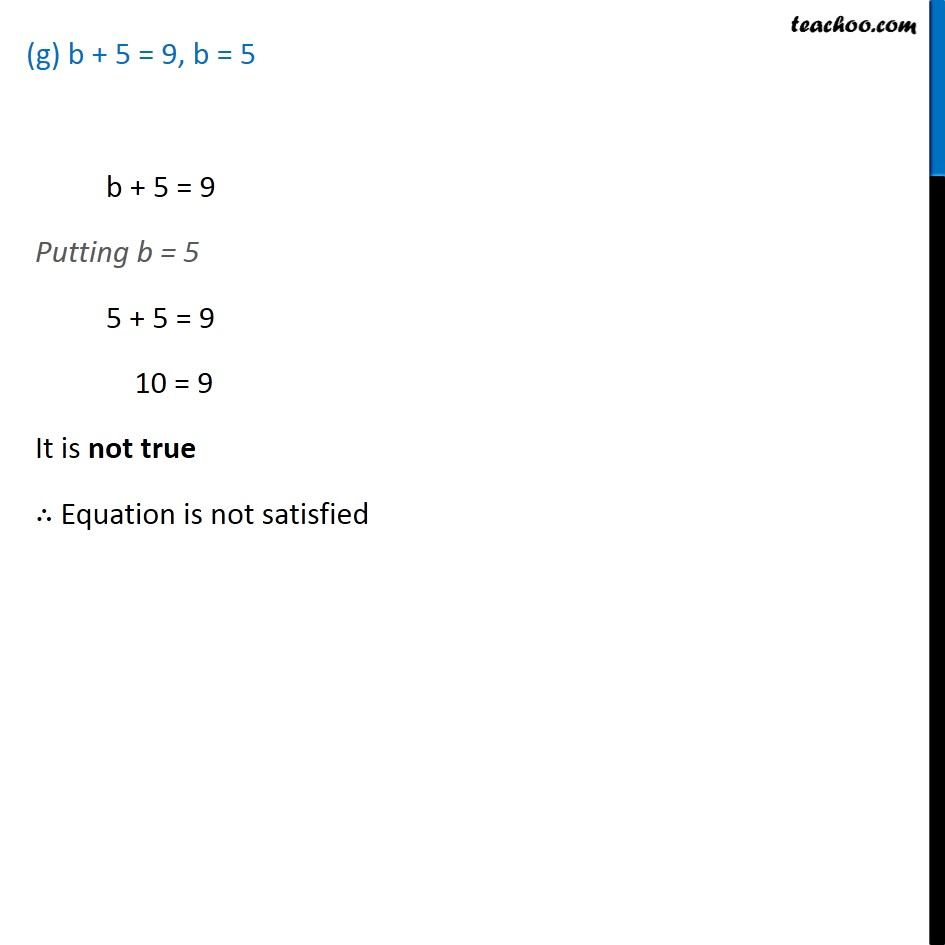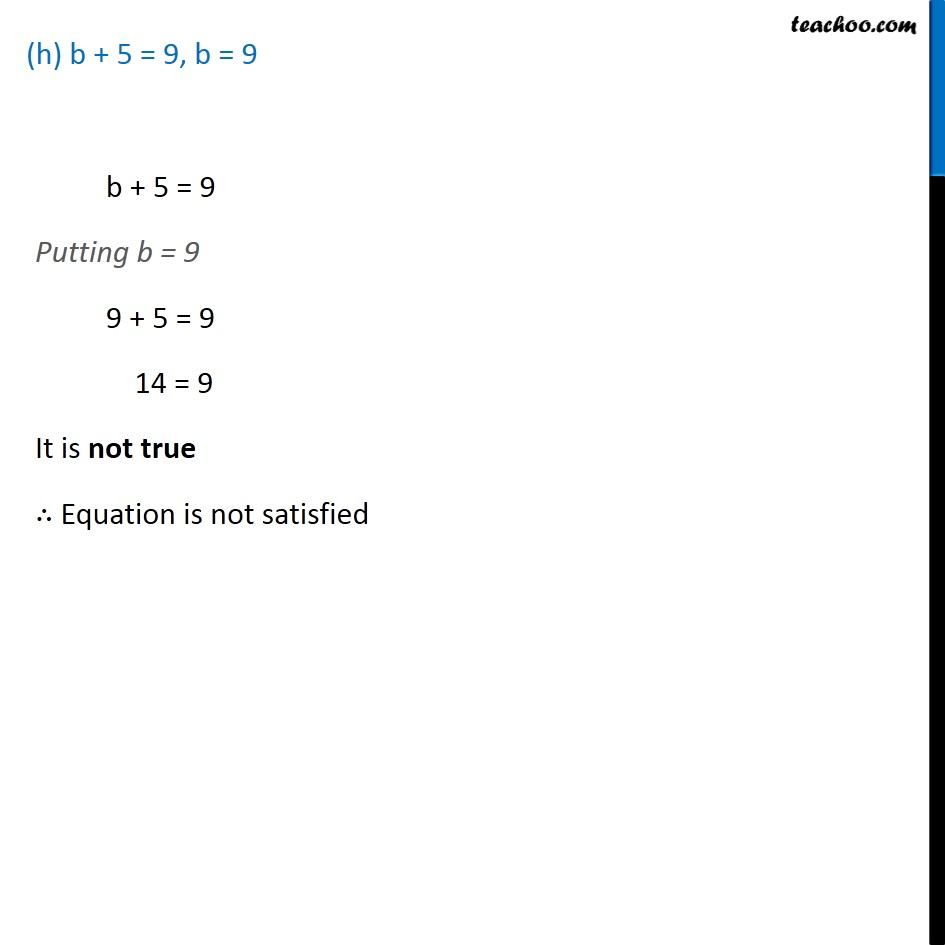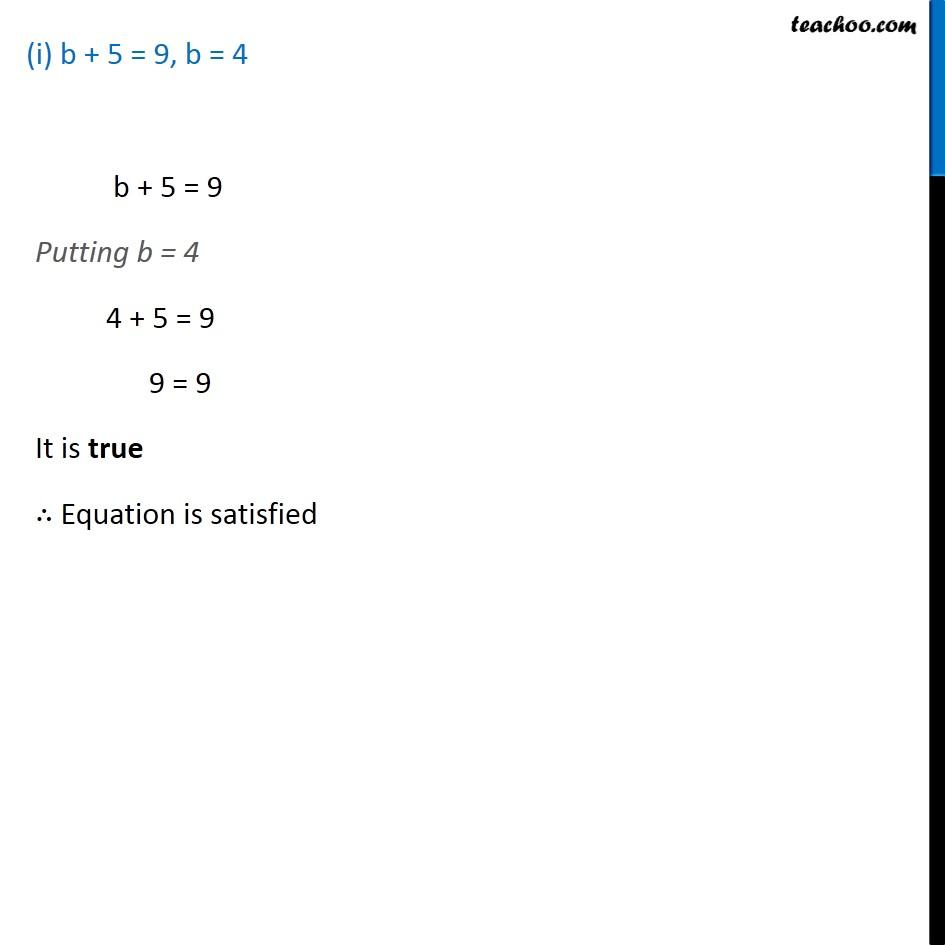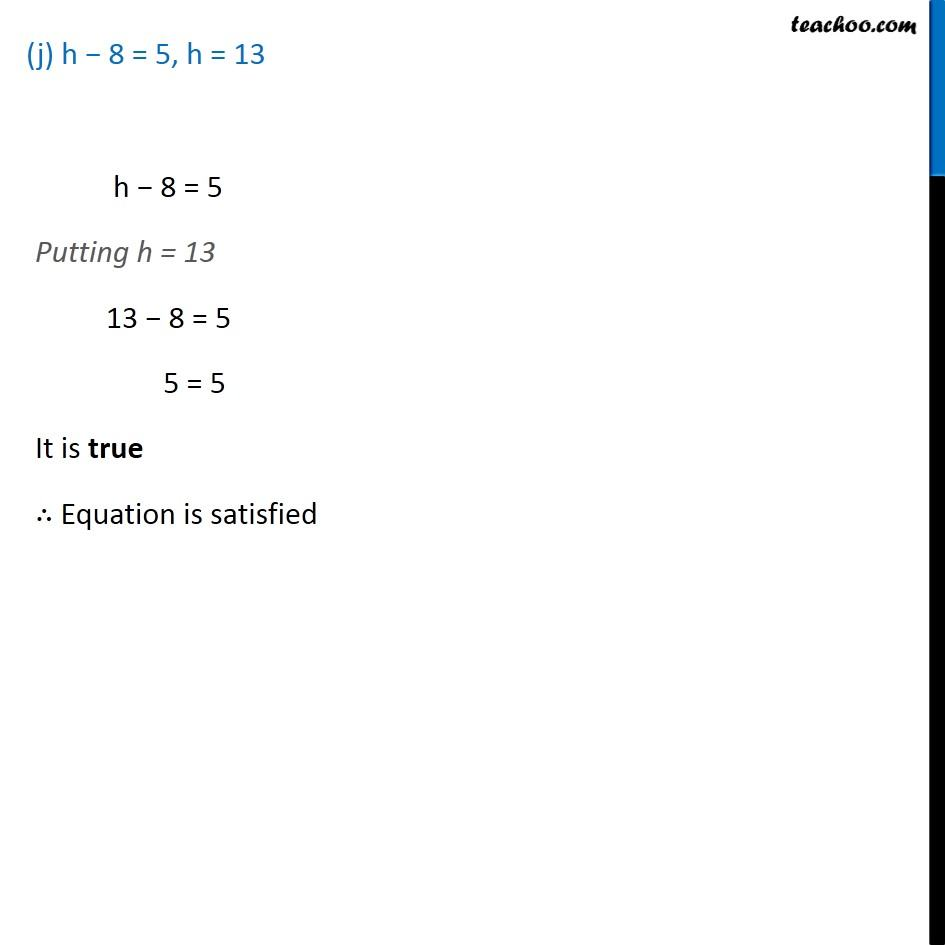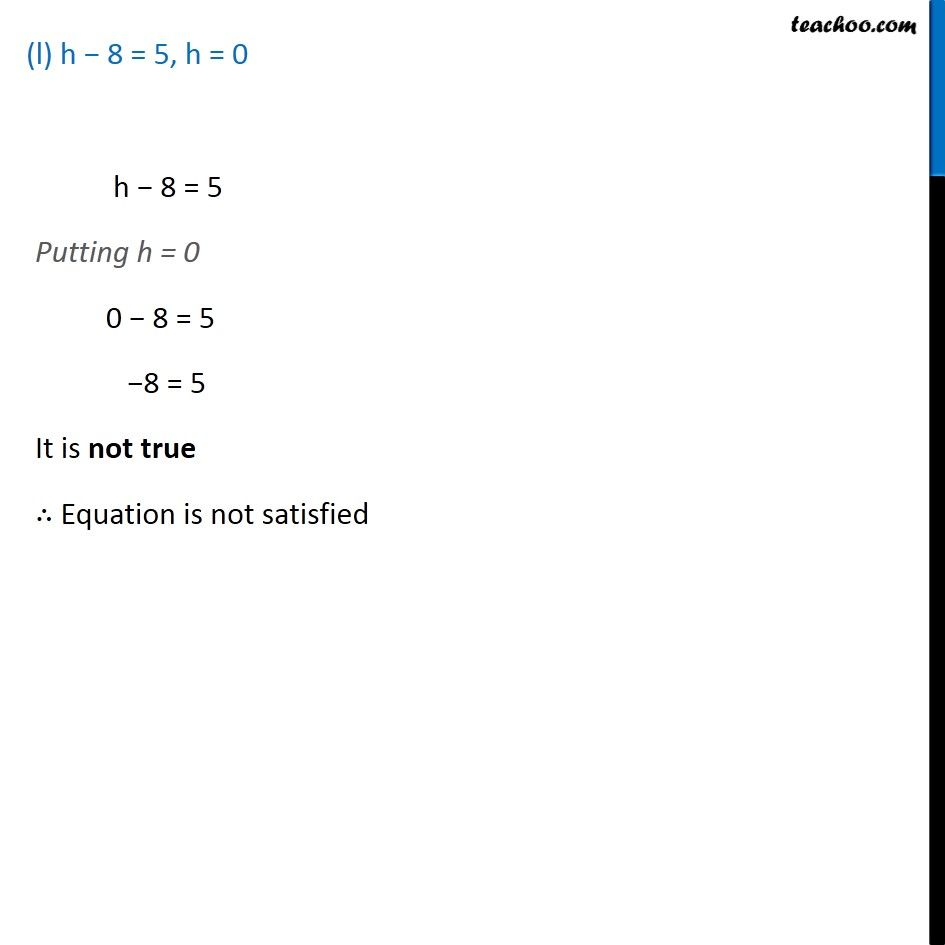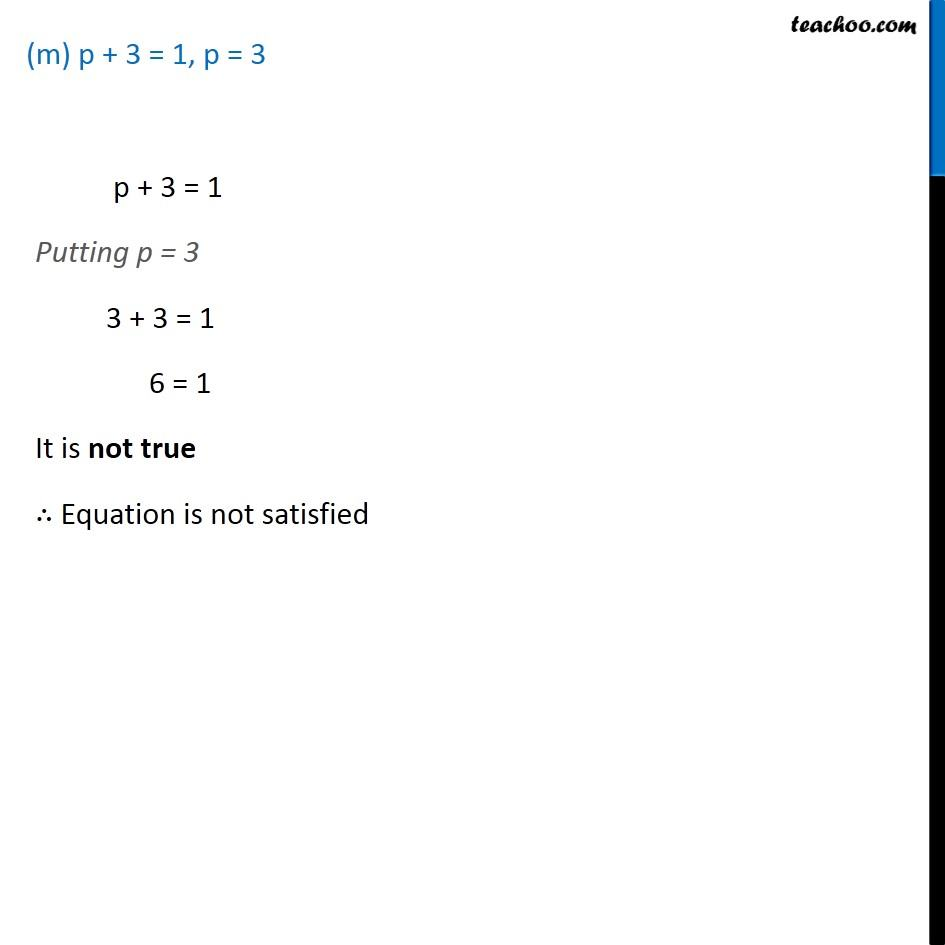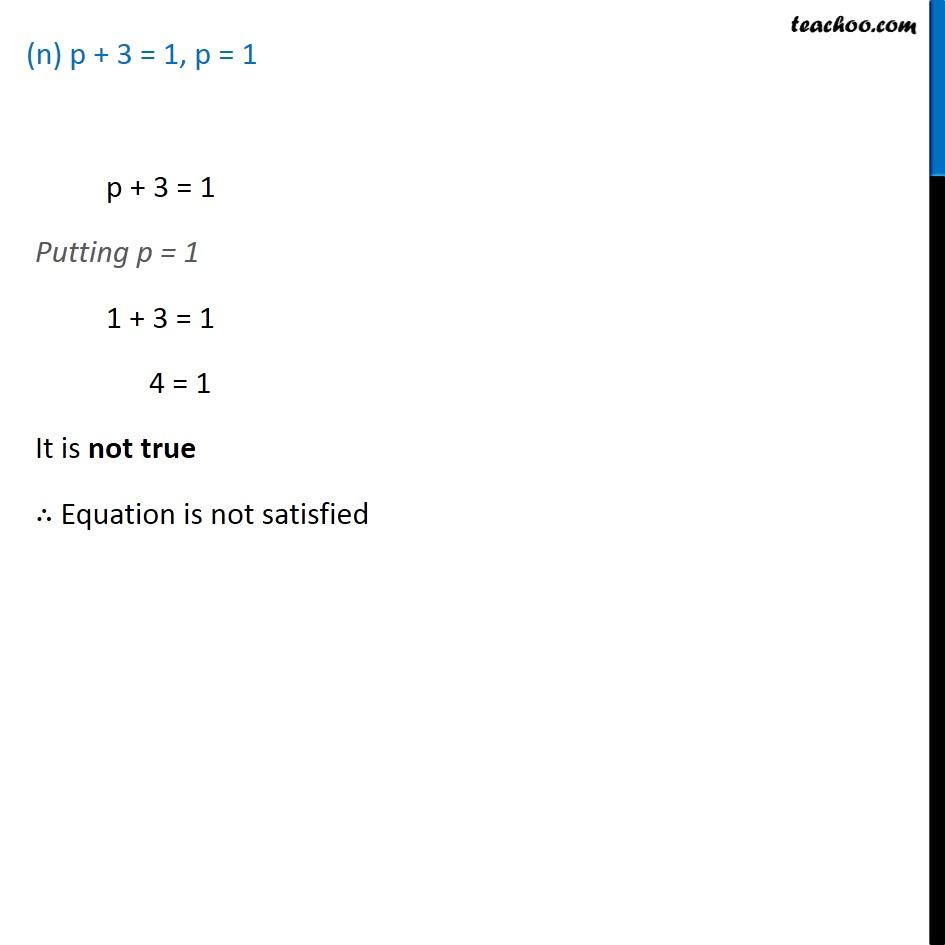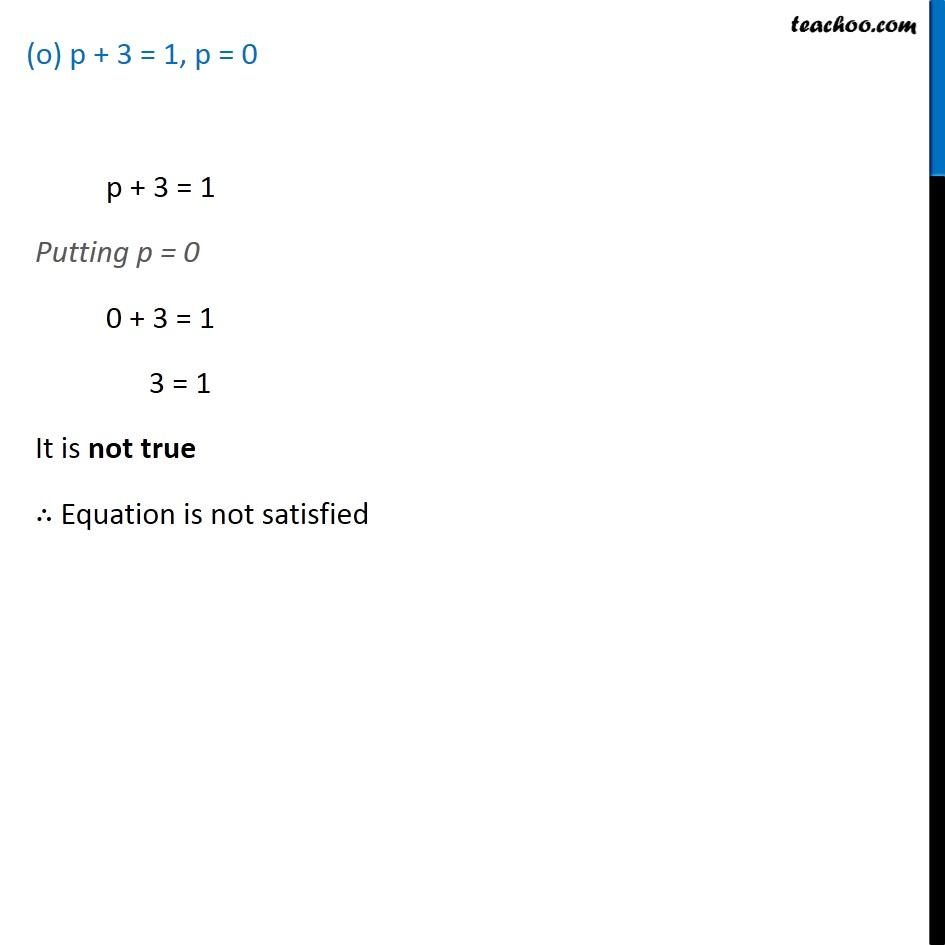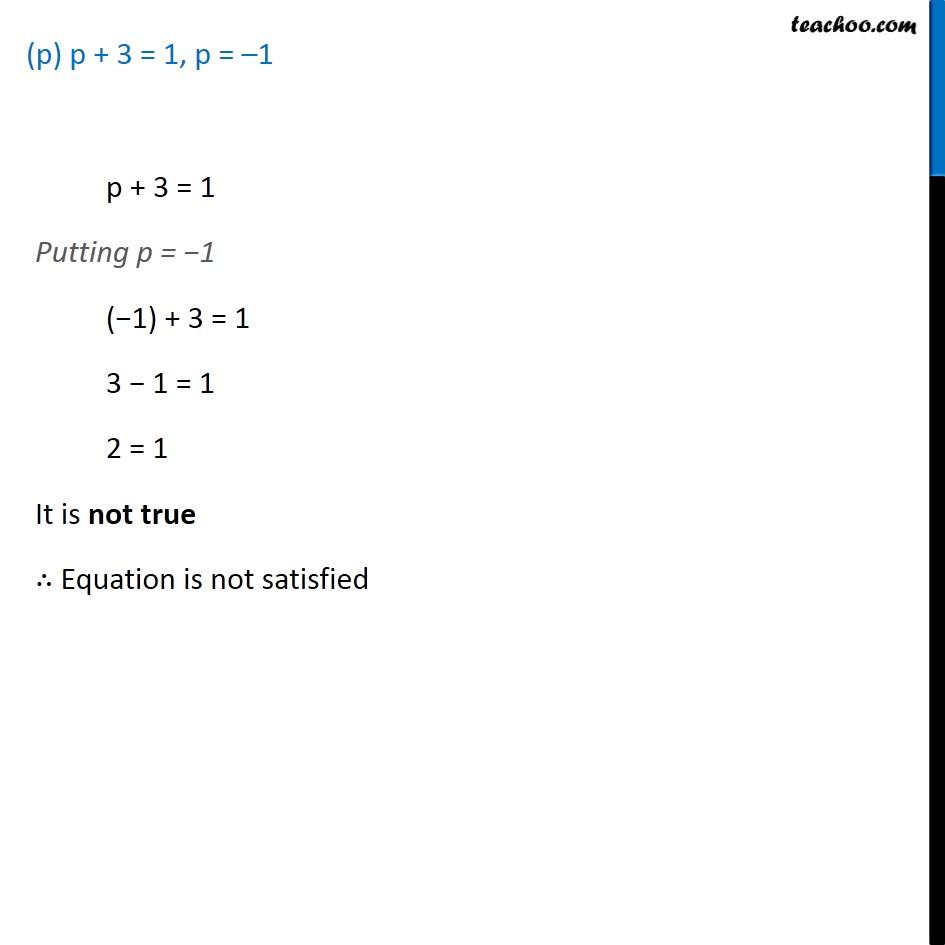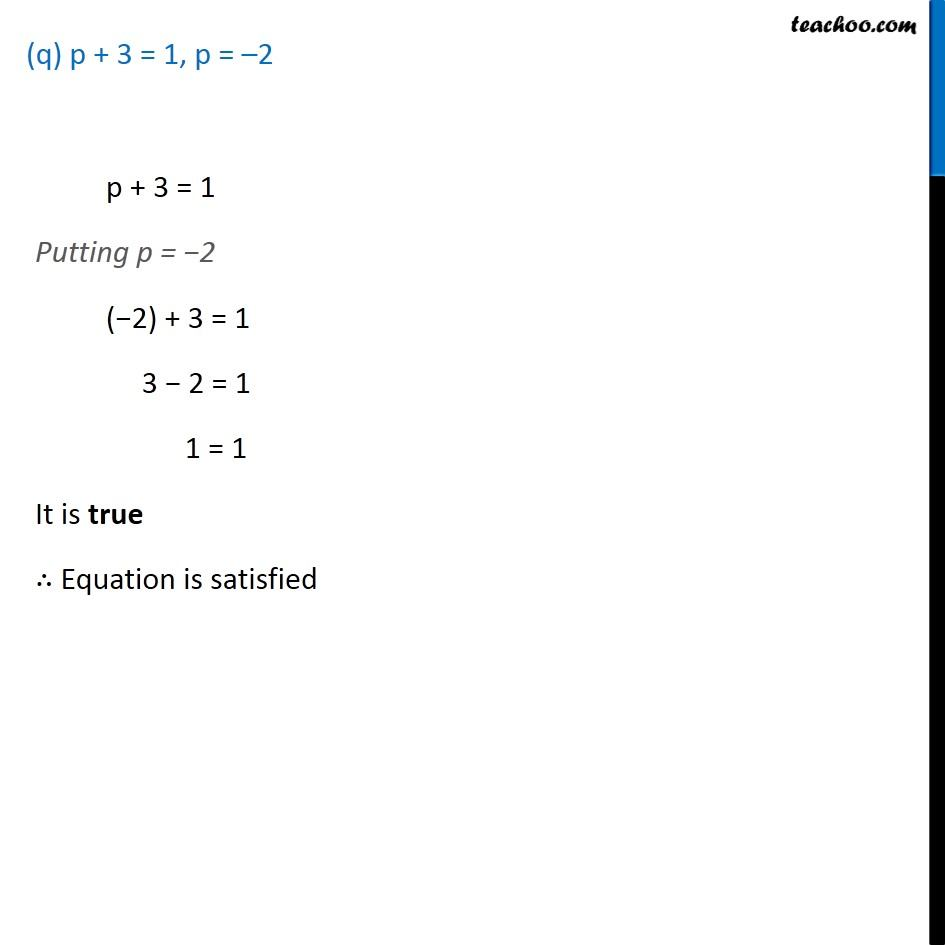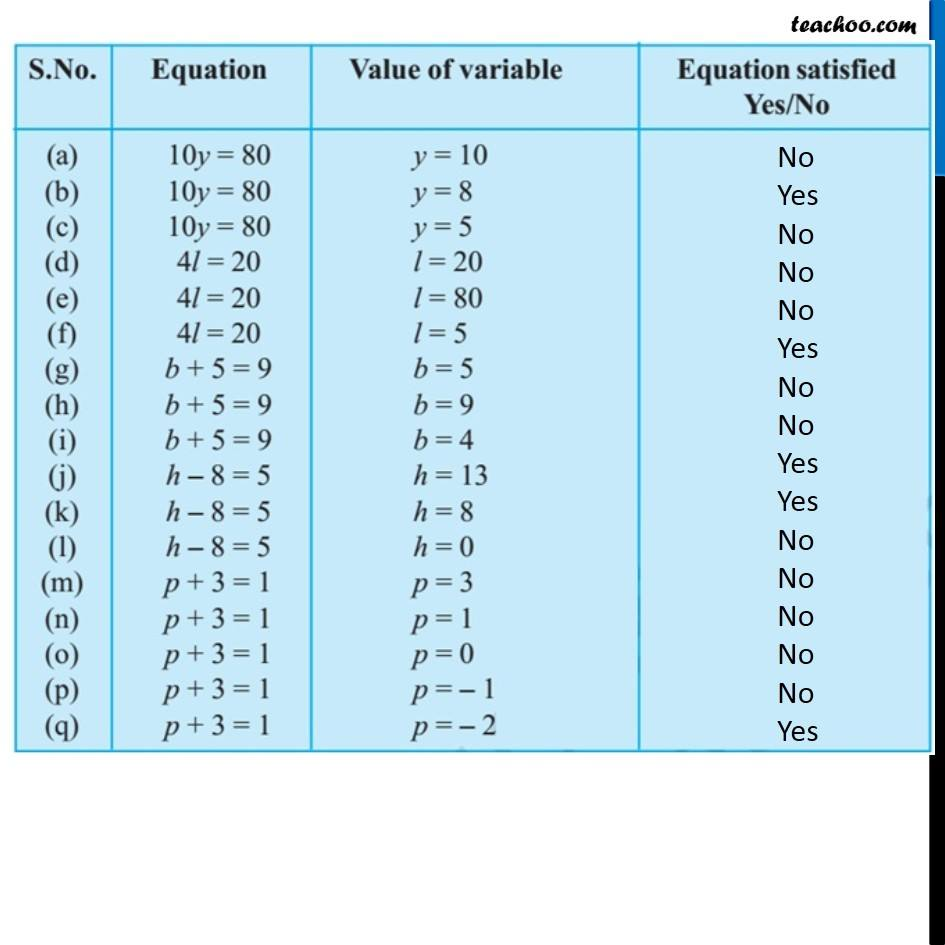1. Chapter 11 Class 6 Algebra
2. Concept wise
3. Equations

Transcript

Ex 11.5, 2 Complete the entries in the third column of the table (a) 10y = 80, y = 10 10 y = 80 Putting y = 10 10 × 10 = 80 100 = 80 It is not true ∴ Equation is not satisfied (b) 10 y = 80 , y = 8 10 y = 80 Putting y = 8 10 × 8 = 80 80 = 80 It is true ∴ Equation is satisfied (c) 10 y = 80 , y = 5 10 y = 80 Putting y = 5 10 × 5 = 80 50 = 80 It is not true ∴ Equation is not satisfied (d) 4l = 20, l = 204l = 20 Putting l = 20 4 × 20 = 20 80 = 20 It is not true ∴ Equation is not satisfied (e) 4l = 20, l = 80 4l = 20 Putting l = 80 4 × 80 = 20 320 = 20 It is not true ∴ Equation is not satisfied (f) 4l = 20, l = 5 4 l = 20 Putting l = 5 4 × 5 = 20 20 = 20 It is true ∴ Equation is satisfied (g) b + 5 = 9, b = 5 b + 5 = 9 Putting b = 5 5 + 5 = 9 10 = 9 It is not true ∴ Equation is not satisfied (h) b + 5 = 9, b = 9 b + 5 = 9 Putting b = 9 9 + 5 = 9 14 = 9 It is not true ∴ Equation is not satisfied (i) b + 5 = 9, b = 4 b + 5 = 9 Putting b = 4 4 + 5 = 9 9 = 9 It is true ∴ Equation is satisfied (j) h − 8 = 5, h = 13h − 8 = 5 Putting h = 13 13 − 8 = 5 5 = 5 It is true ∴ Equation is satisfied (k) h − 8 = 5, h = 8(k) h − 8 = 5, h = 8 (l) h − 8 = 5, h = 0(l) h − 8 = 5, h = 0 (m) p + 3 = 1, p = 3 p + 3 = 1 Putting p = 3 3 + 3 = 1 6 = 1 It is not true ∴ Equation is not satisfied (n) p + 3 = 1, p = 1 p + 3 = 1 Putting p = 1 1 + 3 = 1 4 = 1 It is not true ∴ Equation is not satisfied (o) p + 3 = 1, p = 0p + 3 = 1 Putting p = 0 0 + 3 = 1 3 = 1 It is not true ∴ Equation is not satisfied (p) p + 3 = 1, p = –1 p + 3 = 1 Putting p = −1 (−1) + 3 = 1 3 − 1 = 1 2 = 1 It is not true ∴ Equation is not satisfied p + 3 = 1 Putting p = −1 (−1) + 3 = 1 3 − 1 = 1 2 = 1 It is not true ∴ Equation is not satisfied p + 3 = 1 Putting p = −1 (−1) + 3 = 1 3 − 1 = 1 2 = 1 It is not true ∴ Equation is not satisfied (q) p + 3 = 1, p = –2 p + 3 = 1 Putting p = −2 (−2) + 3 = 1 3 − 2 = 1 1 = 1 It is true ∴ Equation is satisfied

Equations

About the AuthorDavneet Singh
Davneet Singh is a graduate from Indian Institute of Technology, Kanpur. He has been teaching from the past 9 years. He provides courses for Maths and Science at Teachoo.